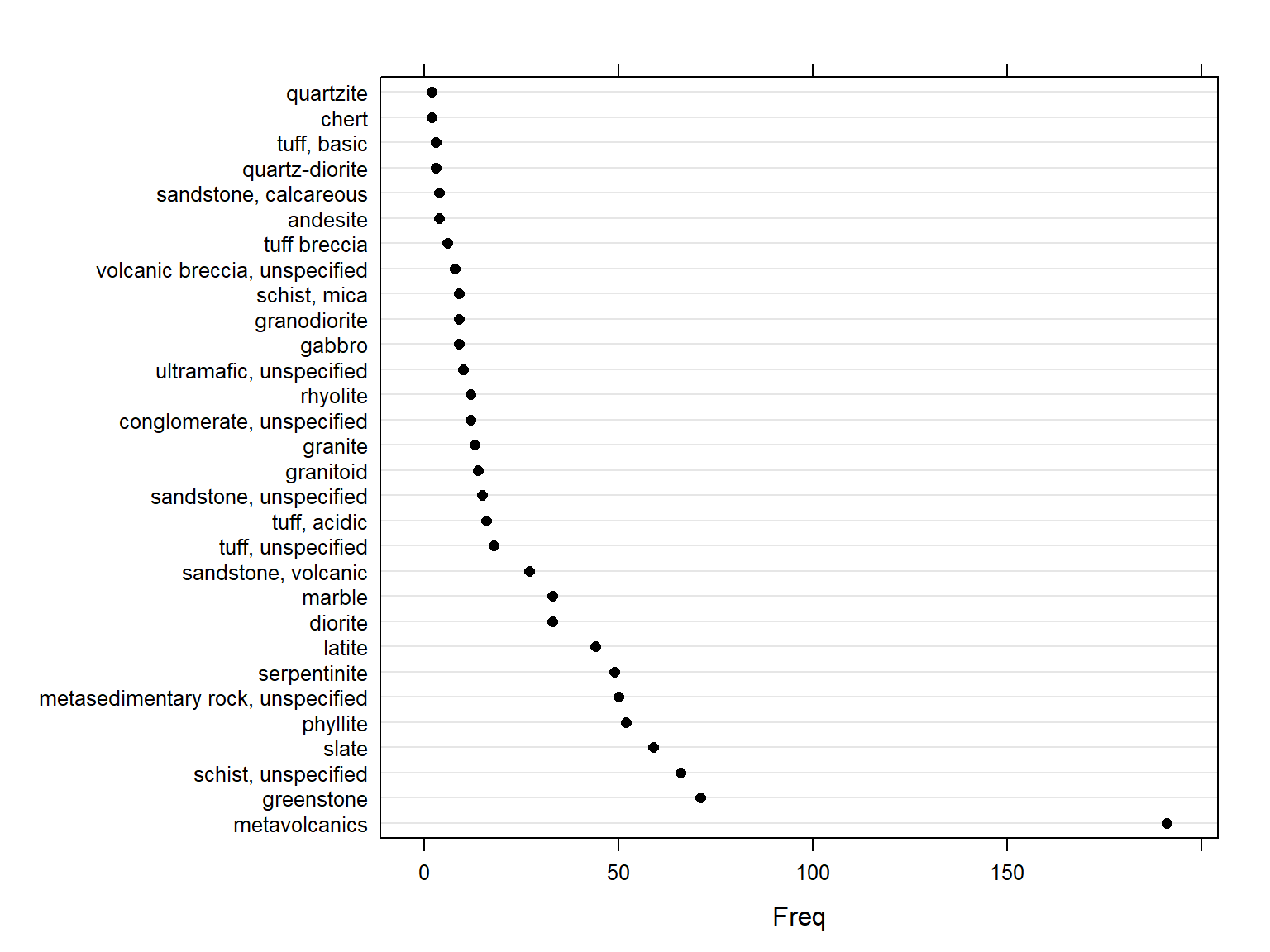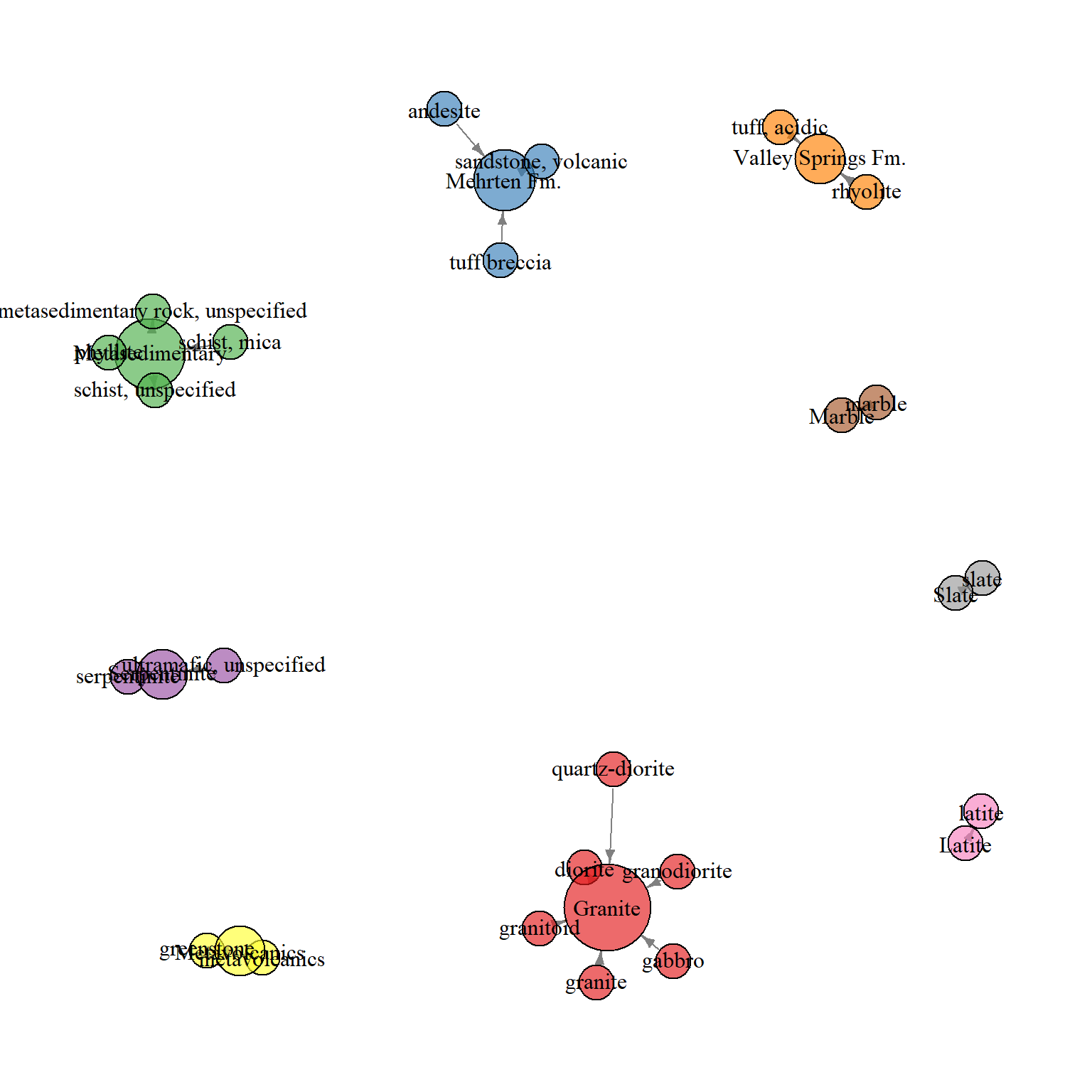## Installation

With a recent version of R, it should be possible to get all of the packages that this tutorial depends on via:

``````# run these commands in the R console
install.packages('aqp', dep=TRUE) # stable version from CRAN + dependencies
install.packages('RODBC', dep=TRUE) # stable version from CRAN + dependencies
install.packages('soilDB', dep=TRUE) # stable version from CRAN + dependencies
install.packages('sharpshootR', dep=TRUE) # stable version from CRAN + dependencies``````

## ODBC Connection to the Local NASIS database

Setting up this connection is simple, and outlined in this guide. Queries submitted to the national database will load pedons or DMU data into your local database. Functions in `soilDB` can only access data in your local database, defined by the "selected set". While it is possible to filter pedon or DMU records within R, it is sometimes simpler to modify the "selected set" in NASIS. In other words:

2. build your selected set (pedon, site, DMU, etc.)
3. load data into R for further filtering/processing

Before running this code, be sure to read the instructions above on setting up and ODBC connection and selected set. This approach could also be used to load equivalent data from a PedonPC database using `fetchPedonPC('path.to.your.data.base.accdb')`. Note that the filtering step described below should be adjusted to fit your data. In this case, we are keeping pedons from the "CA630" survey area, and filtering based on the occurrence of this pattern within the user site ID.

``````# load required libraries
library(soilDB)
library(lattice)
library(sharpshootR)
library(igraph)

# setup plotting style for later
tps <- list(superpose.line=list(col='RoyalBlue', lwd=2))

# load all pedons from the selected set, skip filterintg of hz errors
f <- fetchNASIS(from='pedons', rmHzErrors=FALSE)

# keep only thermic STR
f <- f[which(f\$taxtempregime == 'thermic'), ]``````

## Tabulate occurrence of various bedrock kinds

The `table()` function is a great way to count the number of cases of each level of a category, or, cross-tabulation among several categories. Objects returned by `table()` can be readily visualized using the `dotplot()` function.

``````# tabulate all bedrock kinds
bt <- table(f\$bedrckkind)

# display just the top 20
dotplot(sort(bt, decreasing=TRUE)[1:30], col='black')``````There are several bedrock kinds that are functionally similar within CA630, so we can group them into a several "generalized bedrock kinds". Note that we are matching bedorock kind with several possible options using the `%in%` operator.

``````# make a new column to store generalized geology, and fill with NA
f\$generalized_bedrock <- rep(NA, times=length(f))

# generate new labels for bedrock kinds that are functionally similar
f\$generalized_bedrock[grep('metavolcanic|greenstone', f\$bedrckkind, ignore.case = TRUE)] <- 'Metavolcanics'
f\$generalized_bedrock[grep('diorite|granite|granodiorite|granitoid|gabbro', f\$bedrckkind, ignore.case = TRUE)] <- 'Granite'
f\$generalized_bedrock[grep('schist|phyllite|metasedimentary', f\$bedrckkind, ignore.case = TRUE)] <- 'Metasedimentary'
f\$generalized_bedrock[grep('slate', f\$bedrckkind, ignore.case = TRUE)] <- 'Slate'
f\$generalized_bedrock[grep('serpentinite|ultramafic', f\$bedrckkind, ignore.case = TRUE)] <- 'Serpentinite'
f\$generalized_bedrock[grep('volcanic breccias|tuff breccia|sandstone, volcanic|andesite', f\$bedrckkind, ignore.case = TRUE)] <- 'Mehrten Fm.'
f\$generalized_bedrock[grep('tuff, acidic|tuff, welded|rhyolite', f\$bedrckkind, ignore.case = TRUE)] <- 'Valley Springs Fm.'
f\$generalized_bedrock[grep('marble', f\$bedrckkind, ignore.case = TRUE)] <- 'Marble'
f\$generalized_bedrock[grep('latite', f\$bedrckkind, ignore.case = TRUE)] <- 'Latite'``````

For now, lets only aggregate those pedons that we have included within the new generalized bedrock classes. For more information on how this process works, see `?subsetProfiles`.

``````# subset only those pedons with generalized bedrock
f.sub <- subsetProfiles(f, s="!is.na(generalized_bedrock)")

# re-tabulate number of pedons / generalized bedrock kind
(bedrock.tab <- table(f.sub\$generalized_bedrock))``````
``````##
##            Granite             Latite             Marble        Mehrten Fm.    Metasedimentary
##                 81                 44                 33                 37                177
##      Metavolcanics       Serpentinite              Slate Valley Springs Fm.
##                262                 59                 59                 28``````

Check cross-tabulation of original / generalized bedrock within subset via graph.

``````tab <- table(f.sub\$bedrckkind, f.sub\$generalized_bedrock)
# convert contingency table -> adj. matrix
# plot using a function from the sharpshootR package
par(mar=c(1,1,1,1))
plotSoilRelationGraph(m, graph.mode = 'directed', edge.arrow.size=0.5)``````## Aggregate select soil properties by depth, within each generalized bedrock kind

See `?slab` for details on how to use this function. Note that this approach combines pedons of all depth-classes. Depending on the number of pedons in the collection, it may take a couple of minutes to finish.

``````## aggregate properties by generalized bedrock kind
a <- slab(f.sub, generalized_bedrock ~ clay + total_frags_pct + phfield)

# compute mid-point between slice top and bottom depth for plotting
a\$mid <- with(a, (top+bottom)/2)

# append the number of pedons to the bedrock label
a\$generalized_bedrock <- factor(a\$generalized_bedrock, levels=names(bedrock.tab), labels=paste(names(bedrock.tab), ' (', bedrock.tab, ')', sep=''))``````

## Plot aggregate data

Lattice graphics are used to plot the median property bounded by the 25th and 75th percentiles, with data split into panels according to generalized bedrock type. Percentages printed on the right-hand side of each panel describe the fraction of pedons contributing data at each depth. See `?panel.depth_function` for details on how to plot aggregate soils data.

``````p.clay <- xyplot(mid ~ p.q50 | generalized_bedrock, upper=a\$p.q75, lower=a\$p.q25, data=a, ylim=c(180,-5), ylab='Depth (cm)', xlab='% Clay', strip=strip.custom(bg='yellow'), as.table=TRUE, panel=panel.depth_function, prepanel=prepanel.depth_function, scales=list(y=list(tick.number=7, alternating=3), x=list(alternating=1)), subset=variable == 'clay', layout=c(9,1), cf=a\$contributing_fraction, sync.colors=TRUE, alpha=0.33)

p.rf <- xyplot(mid ~ p.q50 | generalized_bedrock, upper=a\$p.q75, lower=a\$p.q25, data=a, ylim=c(180,-5), ylab='Depth (cm)', xlab='% Frags', strip=strip.custom(bg='yellow'), as.table=TRUE, panel=panel.depth_function, prepanel=prepanel.depth_function, scales=list(y=list(tick.number=7, alternating=3), x=list(alternating=1)), subset=variable == 'total_frags_pct', layout=c(9,1), cf=a\$contributing_fraction, sync.colors=TRUE, alpha=0.33)

p.ph <- xyplot(mid ~ p.q50 | generalized_bedrock, upper=a\$p.q75, lower=a\$p.q25, data=a, ylim=c(180,-5), ylab='Depth (cm)', xlab='pH', strip=strip.custom(bg='yellow'), as.table=TRUE, panel=panel.depth_function, prepanel=prepanel.depth_function, scales=list(y=list(tick.number=7, alternating=3), x=list(alternating=1)), subset=variable == 'phfield', layout=c(9,1), cf=a\$contributing_fraction, sync.colors=TRUE, alpha=0.33)``````

## Apply plot style and combine figures into a single composite

``````# apply styling/colors
trellis.par.set(tps)

# combine sub-plots
print(p.clay, split=c(1,1,1,3), more=TRUE)
print(p.rf, split=c(1,2,1,3), more=TRUE)
print(p.ph, split=c(1,3,1,3), more=FALSE)``````